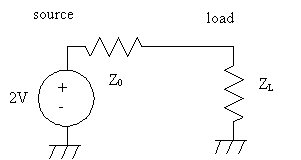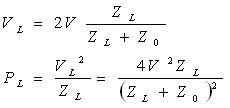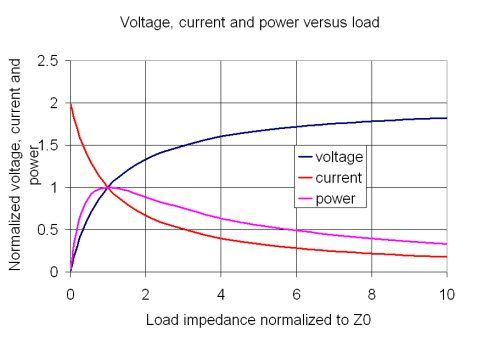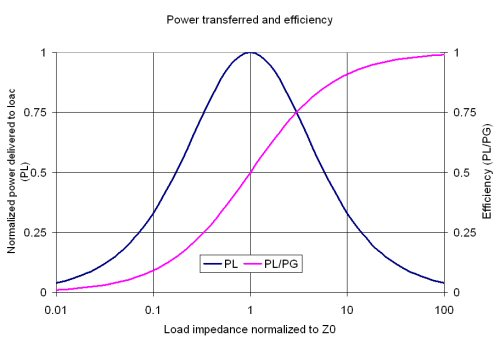# Maximum Power Transfer Theorem

The importance of matching a load to a source for maximum power transfer is extremely important in microwaves, as well as all manner of lower frequency stuff such as stereo sound systems, electrical generating plants, solar cells and hybrid electric cars. It is very simple to prove using Ohm's Law, and that's what we'll do here.

The first engineer to understand the importance of source and load matching was American Joseph Henry. Check out his portrait in our Microwave Hall of Fame!

The maximum power transfer theorem is sometimes called the conjugate match thoerem.  Neophytes frequently misapply the thoerem to situations where it is not appropriate. iI is useful only for designing loads, not generators.

A circuit diagram of a source and load is shown below. Feel free to think of everything as direct current because that is the simplest case (RF follows ohm's law, so the theory is valid at any frequency). The source has a series resistance (often called the Norton or Thevenin equivalent resistance) built into it. In microwave engineering, the generator's resistance is the same as the characteristic impedance of the transmission media, usually 50 ohms, and it is usually called "Z0" (sometimes ZC for "characteristic impedance". The load resistance is called ZL.Notice that the generator voltage is "2V" in the schematic. Some people are wondering, "if I buy a 1.5 volt D-cell battery, does that mean that it actually can put out 3 volts?" No way, Jose! What we are trying to show here is that under maximum power transfer conditions, only half of the generator voltage makes its way to the load... so in the case of a D-cell, only 0.75 volts would be available at maximum power transfer. Then you ask, "why doesn't maximum power transfer happen when I put a short circuit on the output, I know that the battery will discharge quickly under that condition?" The problem here is that total dissipation will be maximum with a short circuit load, but no power will be transferred out of the battery. All of the power dissipation is internal to the battery, which is why it is getting too hot to hold....

Using Ohm's law we can solve for the transferred power. First note that Z0 and ZL form a voltage divider. Then recall that power is equal to voltage squared divided by resistance:Now let's look at output voltage, current and as a function of load resistance. For convenience we will normalize the load resistance to the characteristic impedance and let them both equal 1; for ZL=twice Z0, the normalized load resistance is 2.0 for example. We further normalize the generator voltage 2V to 2 volts.The equation for power, plotted in purple, has a funny shape with a clear-cut maximum. For homework you can use calculus to find the maximum, but trust us (and the plot), it occurs at exactly (ZL/ZC)=1!

We can see from the plot, the maximum output current occurs into a normalized impedance of zero (a short circuit). Here we get a DC current of 2, which is because the generator has a voltage of 2. Looking at an open circuit (off to the right of the plot) we can see that the load voltage asymptotically approached 2 volts. By multiplying the current and voltage, power is obtained.

Under open-circuit conditions (ZL=infinity) the output voltage is "2V". Under short-circuit conditions, a current of 2V/Z0 would result.

Going back to our D-battery analogy, how can you find the source impedance of a battery? You could put a variable load on it, and when the load voltage hits 0.75 volts, it would have the same value as the generator impedance. A faster way is to measure the short circuit current (quickly!), then divide the open circuit voltage (1.5 volts) by the short circuit current (perhaps three amps) and you will arrive at the generator impedance of maybe 0.5 ohms.

Now lets look at efficiency versus load impedance. This time we'll plot a wide range of load impedances using a log scale. The log scale chart is interesting from the point of view of the symmetries involved. In addition to the maximum power point at RL/RG=1 providing 50% efficiency, the point at which RL/RG=3 provides exactly 75% efficiency. One lesson you could take away from this is that for highest efficiency, the load impedance must be much greater than the generator impedance even though maximum power transfer will not take place under this condition. In the two extreme condictions, a short circuit is 0% efficient, and open circuit is 100% efficient, even though in both cases, no power is transferred to the load.By analogy, for a current source the efficiency increases with GL/GG. In this case a diminishing load resistance leads to higher efficiency. The two types of sources have opposite behaviors as far as efficiency relative to load resistance is concerned.

### What does this say about amplifiers?

Regarding power amplifier efficiency, varying the slope of the load line in Class A does not have such a predictable effect on efficiency. For amplifiers in or near compression power and efficiency are complex functions of many factors including the outermost excursions of the dynamic loadline near the pinch-off area and near the Imax boundary. Too much of a topic to squeeze onto this one page!

Author : Unknown Editor